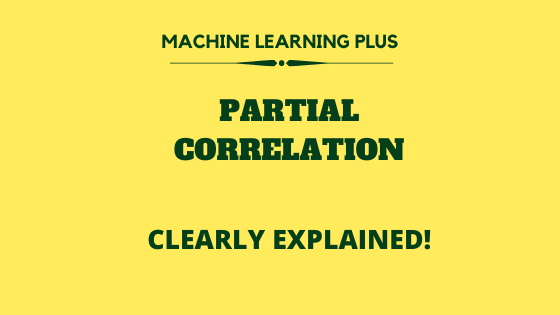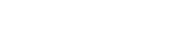# Partial Correlation## What is Partial Correlation and it’s purpose

Partial correlation is used to find the correlation between two variables (typically a dependent and an independent variable) with the effect of other influencing variables being controlled.

For example, if there are three variables ‘A’, ‘B’, ‘Z’, If you want to find the relationship between ‘A’ and ‘B’ with the influence of ‘Z’ being controlled you can use partial correlation.

It is useful in several situations in real world, and can enrich your EDA results with more valuable insights.

Related: Complete Data Preprocessing and EDA course by Selva (Principal Data Scientist)

## Difference between Simple Correlation and Partial Correlation

Simple correlation (a.k.a. Pearson correlation coefficient) may not give a complete picture while trying to understand the relationship between two variables (A and B) especially when there exist other influencing variables that affect A (and/or) B.

In fact, simple correlation mainly focuses on finding the influence of each variable on the other.

Whereas partial_correlation is used to find the refined relationship between two variables with the effect of the other influencing variables being excluded/controlled.

Let’s look at some examples where you can use Partial correlation.

## Example of Partial Correlation in real world

1) Education: If you have three variables study hours, marks obtained, classes attended, and want to find the correlation between the classes attended and marks obtained by controlling the effects of study hours. Partial correlation will be relevant here because ‘study hours’ might be dependent on the classes attended (and marks) as well and you might want to see the pure relationship between these two, excluding the effect of study hours.

2) Weather Detection: If you have three variables aerosol particles and abundance of cloud and wind speed. You can use partial correlation to find the relationship between the amount of aerosol and the abundance of clouds.

3) Weight Detection: The variables can be quantity of food, weight increase, calories. This technique can be used to find the relationship between the quantity of food, weight increase, and the variable being controlled is calories.

## Creating the dataset and visualization

# Create a sample dataset
import pandas as pd
import matplotlib.pyplot as plt
import math
Data = {'A' : [4, 2, 2, 1, 8, 6, 9, 8, 11, 13, 12, 14],
'B' : [1, 2, 2, 4, 9, 8, 9, 6, 14, 12, 13, 12],
'Z' : [1, 2, 3, 4, 1, 2, 3, 4, 1, 2, 3, 4]}
df = pd.DataFrame(Data, columns = ['A', 'B', 'Z'])
df


Let’s create a scatterplot of the variables ‘A’ and ‘B’

# Scatterplot to understand the relationship
plt.plot(df["A"],df["B"],'ro')
plt.xlabel("A")
plt.ylabel("B")


Clearly, as ‘A’ increases, ‘B’ also increases.

Let’s calculate the Pearson correlation first before calculating Partial correlation.

#### Get Free Complete Python Course

Facing the same situation like everyone else?

Build your data science career with a globally recognised, industry-approved qualification. Get the mindset, the confidence and the skills that make Data Scientist so valuable.#### Get Free Complete Python Course

Build your data science career with a globally recognised, industry-approved qualification. Get the mindset, the confidence and the skills that make Data Scientist so valuable.

# Calculate pearson correlation
df.corr()


## Partial Correlation Calculation

The pingouin has a function called .partial_corr to calculate the partial_correlation.

#!pip install pingouin
import pingouin as pg
pg.partial_corr(data=df, x='A', y='B', covar='Z')

# Where,
# Data = Name of the dataframe.
# x  = Name of column in dataframe.
# y = Name of column in dataframe.
# z = variable to be excluded/controlled.


The partial correlation value we get after excluding ‘Z’ is 0.910789 which corresponds to a strong positive correlation. To calculate the partial_correlation between multiple variables .pcorr() function is used.

df.pcorr().round(7)


In this case, the Partial correlation is coming out to be greater than the Pearson correlation. This can be the case when the 3rd variable is having a negative correlation relationship with one of the variables.

Otherwise, typically, the Partial correlation is lesser than Pearson correlation.

## Limitations of Partial correlation

Some limitations of partial_correlation analysis are:

1. The calculation of partial_correlation totally depends on the simple correlation coefficient. Simple correlation coefficient assumes relationships to be in linear form. But in real world the phenomena of linear relationships are quite rare.
2. When the order of partial_correlation coeffcient increases, its reliability decreases.

3. Its calculation are quite difficult i.e.finding the value of ‘r’ can be quite difficult and time consuming. But things are quite easy because there are so many softwares and libraries available to perform this job.

4. As the number of controlling variables increases, calculating partial_correlation will get more complicated.

## Similar Articles

### Logistic Regression – A Complete Tutorial With Examples in RCourse Preview

## Machine Learning A-Z™: Hands-On Python & R In Data Science

### Free Sample Videos:#### Machine Learning A-Z™: Hands-On Python & R In Data Science#### Machine Learning A-Z™: Hands-On Python & R In Data Science#### Machine Learning A-Z™: Hands-On Python & R In Data Science#### Machine Learning A-Z™: Hands-On Python & R In Data Science